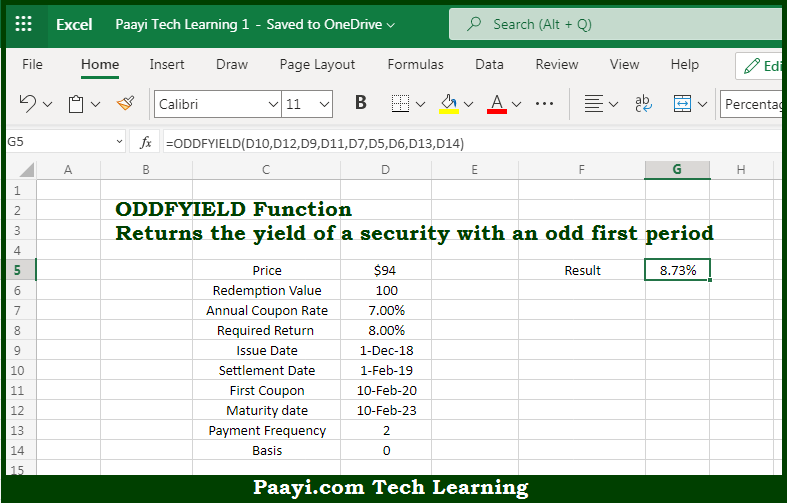# Learn How to Use Microsoft Excel ODDFYIELD Function

Written by | 0 Comments | 587 Views

In this article, you will learn how to use the Microsoft Excel ODDFYIELD function and its prime function in Microsoft Excel. You will also get to know the Microsoft Excel ODDFYIELD function return value and syntax with the help of some examples.

Microsoft Excel ODDFYIELD Function

The main purpose of the Microsoft Excel ODDFYIELD function is to get the yield security with a first odd period. That implies, with the help of the ODDFYIELD function you can able to return the yield of a security with an odd (irregular) first period. So, with the help of the ODDFYIELD function, you can able to get the yield security with a first odd period.

Return Value of ODDFYIELD Function

The return value will be yield as a percentage.

Syntax of ODDFYIELD Function

=ODDFYIELD(sd, md, id, fd, rate, pr, redem, freq, [basis])

Where the arguments:

• sd - This is the settlement date of the security.
• md - This is the maturity date of the security.
• id - This is the issue date of the security.
• fd - This is the date of the first coupon.
• rate - This is the annual coupon rate of security.
• pr - This is the price of the security.
• redem - This is the redemption value per \$100 face value.
• freq - This is the coupon payments per year, where, annual = 1, semiannual = 2; quarterly = 4.
• basis - This is the day count basis, that's defaults to 0 (optional).

How to Use Microsoft Excel ODDFYIELD Function?So we know that Microsoft Excel ODDFYIELD function you can able to get the yield security with the first odd period. That implies, with the help of the ODDFYIELD function you can able to return the yield of a security with an odd (irregular) first period. So, with the help of the ODDFYIELD function, you can able to get the yield security with the first odd period.